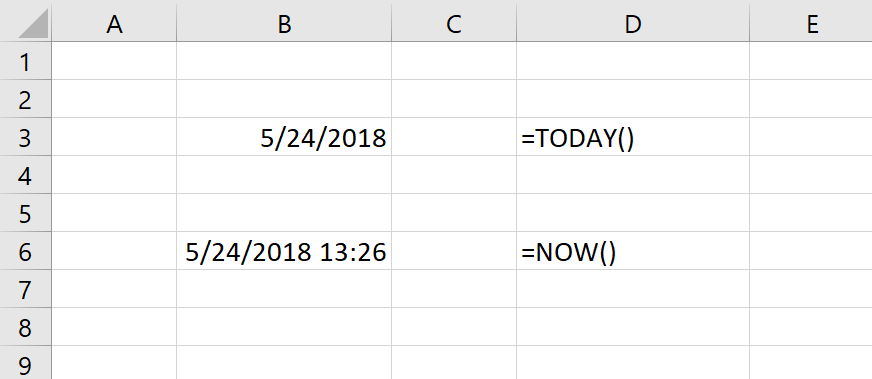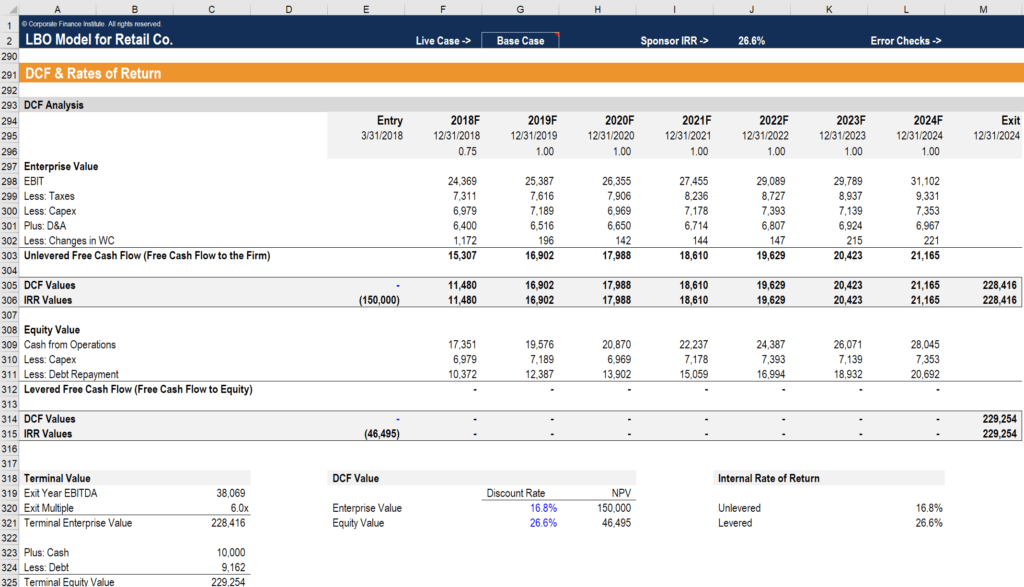# Excel Current Date and Time

Insert the current date and time into an Excel spreadsheet

## How to insert the current date and time in Excel?

For anyone working as a financial analyst, it can be useful to insert the current time and date into an Excel spreadsheet. This guide will break down how the Excel current date and time function works and outline situations where it will be helpful in your analysis.

### Excel current date and time formulas (dynamic)

There are two formulas to use, depending on what type of information you’re looking to insert in your spreadsheet. Note: These are dynamic formulas and will update whenever a spreadsheet is opened.

Current date formula:

=TODAY()

Current time formula:

=NOW()

### Excel current date and time example

Let’s look at a real example in an Excel spreadsheet of how these two formulas work. In the screenshot below, you can clearly see how each works and what the corresponding output is. For example, if, at the time of creating the formula, it’s May 24, 2018 at 1:36 p.m., then the following information will appear in your spreadsheet. NOTE: Excel uses a 24-hour clock when it outputs the time.As you can see, the =TODAY() formula only includes the day, month and year. The =NOW() function displays more information, showing the day, month, year, hour and minutes (using a 24-hour clock).

### Excel current date and time formulas (static)

You may not always want the figures in the file to update every time you open the file. If this is the case, then you’ll want to insert a static version of the formulas.

Static formulas are:

• “Ctrl + ;” – inserts the date (Windows)
• “Ctrl + Shift + ;” – inserts the date and time (Windows)
• “COMMAND + ;” (Mac)

### Why insert current date and time in Excel?

There are many reasons you may want to display the current date and time in Excel. Let’s say that you want users to have the current time displayed on a cover page every time you print off a financial model.

Reasons to include time and date include:

• Creating an activity log
• On a cover page
• When printing a document
• For version control
• When showing time-sensitive information
• When discounting cash flows to the present (Net Present Value and XNPV function)

### How to change the date and time formatting

You may wish to change the format of date or time displayed in the spreadsheet. In order to do this, press F1 (or right-click on the cell and click Format Cells). Once you see the Format Cells box appear on the screen, you can click on Number and then select Date or Time and choose the formatting you want to appear in your spreadsheet.

### Applications in financial modeling

The Excel current time and date function is very useful in performing financial analysis, as time is a critical factor in financial modeling and valuation. The most important use is in discounting cash flows and ensuring that the net present value date is correct. You may wish to use a static or dynamic version of the formulas shown in this article, depending on the analysis being performed.Image from CFI’s financial modeling courses.

Thank you for reading CFI’s guide on inserting the current date and time in Excel. To continue your development as a world-class financial analyst, these additional CFI resources will be helpful:

• Excel for Finance
• Excel Shortcuts for PC & Mac
• List of Excel Functions